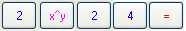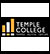Common > How To: Numbering Systems

How To: Numbers © R. Craig Collins, 2005

Why are there different numbering systems? Just as different countries use different words for the same item, there are different numbering systems that represent the same thing in a different way.

Why are numbers important? Computer only really use 1s and 0s, so from a theoretical point of view, you should be able to convert our human base 10 numbers to a computer friendly base 2 number. But from a practical point of view: if you are making web pages, and you don't want to be stuck with 16 word colors, you have to be able to express mixtures of red, green, and blue in numbers. The most widely available graphics program is Paint, but it gives the numerical values of red, green, and blue in base 10... but web pages use base 16, hexadecimal. So, just to be able to use a lot of colors, you need to be able to convert the numbers.

So, you should know the process.
But I know what most of you will do, use a calculator to convert; that's fine, IF you understand the theory.

The three rules of modern numbering systems:

1. Begin with 0 (zero)
2. The base indicates the number of different characters used to represent the numbers
(examples base 10 has 10 characters, base two has 2 characters)
3. When you run out of different characters, add a decimal place to the left, and start over.
 Decimal (base 10) Binary (Base 2) Hexadecimal (Base 16) 0 0 0 1 1 1 2 10 2 3 11 3 4 100 4 5 101 5 6 110 6 7 111 7 8 1000 8 9 1001 9 10 1010 A 11 1011 B 12 1100 C 13 1101 D 14 1110 E 15 1111 F 16 10000 10

You should be able to recreate the above chart.
There is a video available to demonstrate counting. The link is below, at the end of the building a 'powers of 2 number line' discussion.

Click here for an chart that that goes to 3210, and includes base4 and base8.

Notice how large the binary numbers get; we need a way to represent those numbers that takes up less space, and is more distinctive. If you wee to look at the larger example, you can see that base 4, 8, or base 16 are quick ways to represent the same base 2 number with less space. Especially base 16. But decimal, base 10, does not easily fit in this pattern.

Lets find out why.

Look at the numbers in the binary column. Notice the numbers that begin with one, and are followed by nothing or zeros and compare those to the decimal equivalent.

 1 10 100 1000 10000 100000 1 2 4 8 16 32

The decimal values are doubling. This becomes the basis of a quick to build
powers-of-two number line.

 210 29 28 27 26 25 24 23 22 21 20 1024 512 256 128 64 32 16 8 4 2 1

There is a video available on counting, and the Powers of 2 number line here.

This number line is a quick key to converting decimal to binary, and binary to decimal; this is the hardest conversion as the number 10 does not occur on the powers of two number line.

The Harder Stuff, part 1: Converting decimal to binary.

11510 converts to ?2

1. Always build a powers-of-two number line. You dont need to calculate it, just start with 1, then double!
2. Notice the subscript is getting smaller that is our clue to subtract.
3. Computers use 1 to represent success (yes) and 0 to represent failure (no). We will always write our successes and failures (1s and 0s) under the powers-of-two number line.

The largest number on the powers-of-two number line we can subtract from 115 is 64. Put a one under the 64 to represent "yes, I can subtract this," then do the math subtract 115-64= 51

 210 29 28 27 26 25 24 23 22 21 20 1024 512 256 128 64 32 16 8 4 2 1 1

Can we subtract the next number to the right from 51? Yes! Record the success with a 1, and do the math. 51-32 =19

 210 29 28 27 26 25 24 23 22 21 20 1024 512 256 128 64 32 16 8 4 2 1 1 1

Can we subtract the next number to the right from 19? Yes! Record the success with a 1, and do the math. 19-16 =3

 210 29 28 27 26 25 24 23 22 21 20 1024 512 256 128 64 32 16 8 4 2 1 1 1 1

Can we subtract the next number to the right from 3? No! Record the failure with a 0, and continue.

 210 29 28 27 26 25 24 23 22 21 20 1024 512 256 128 64 32 16 8 4 2 1 1 1 1 0

Can we subtract the next number to the right from 3? No! Record the failure with a 0, and continue.

 210 29 28 27 26 25 24 23 22 21 20 1024 512 256 128 64 32 16 8 4 2 1 1 1 1 0 0

Can we subtract the next number to the right from 3? Yes! Record the success with a 1, and do the math. 3-2 =1

 210 29 28 27 26 25 24 23 22 21 20 1024 512 256 128 64 32 16 8 4 2 1 1 1 1 0 0 1

Can we subtract the next number to the right from 1? Yes! Record the success with a 1, and do the math. 1-1 =0

 210 29 28 27 26 25 24 23 22 21 20 1024 512 256 128 64 32 16 8 4 2 1 1 1 1 0 0 1 1

There are no more subtractions possible, but we have our number! 11510 converts to 11100112

Note: since 115 is odd, it must end in 1.

The Harder Stuff, part 2: Converting binary to decimal.

111012 converts to ?10

1. Always build a powers-of-two number line. You dont need to calculate it, just start with 1, then double!
2. Notice the subscript is getting larger that is our clue to add.
3. Computers use 1 to represent success (yes) and 0 to represent failure (no). We will always write our successes and failures (1s and 0s) under the powers-of-two number line.

Write the 1s and 0s under the powers-of-two number line.

 210 29 28 27 26 25 24 23 22 21 20 1024 512 256 128 64 32 16 8 4 2 1 1 1 1 0 1

Add the value above the successes (1s) and ignore the failures (0s).

16+8+4+1 = 29

We have our number! 111012 converts to 2910

The Really Easy Stuff, part 2: Binary to Hexadecimal

1100010111012 converts to ?16

Divide the number into blocks of four, starting from the right. If you dont have enough numbers, add 0s to the left.

1100 0101 1101

Put a short powers-of-two number line above each of the blocks of four; remember, the 1s and 0s always go under the powers-of-two number line.

 8 4 2 1 8 4 2 1 8 4 2 1 1 1 0 0 0 1 0 1 1 1 0 1 12 5 13 12 in hex from the chart , C 5 in hex from the chart , 5 13 in hex from the chart , D

Theres our number! 1100010111012 converts to C5D16

 B A D 11 in hex from the chart or 10 in hex from the chart or 13 in hex from the chart or 1011 1010 1011

1011 1010 1101 2

Just for jollies, Hexadecimal, a BAD numbering system, to Decimal (just use all the skills he have learned)

 B A D 11 in hex from the chart or 10 in hex from the chart or 13 in hex from the chart or 1011 in binary from chart of conversion 1010 in binary from chart of conversion 1101 in binary from chart of conversion

Put 101110101101 under a powers-of-two number line, add the successes to get 298910!

PRACTICE QUESTIONS

Converting decimal to binary.

1310 converts to ?2

25510 converts to ?2

510 converts to ?2

Converting binary to decimal.

10112 converts to ?10

1112 converts to ?10

1110102 converts to ?10

2516 converts to ?2

1F16 converts to ?2

F116 converts to ?2

2516 converts to ?10

1F16 converts to ?10

F116 converts to ?10

See also, a video on Starting the Windows Calculator, or Using the Windows Calculator

First, go to View menu, and choose Scientific View.
In newer versions of Windows, choose Programmer View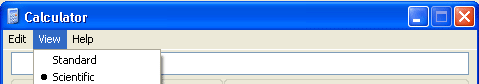Again, in newer versions of Windows, choose Programmer View

If the starting number is in base 10, set the button to Dec,
enter the number to convert,
then click to bin, oct, or hex.

Example 12810=?16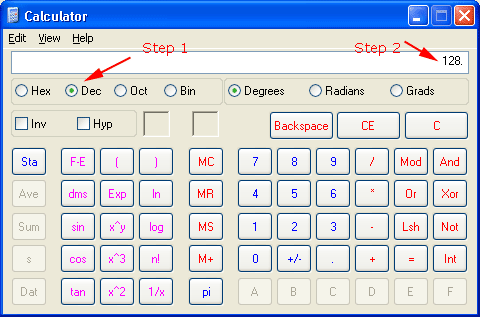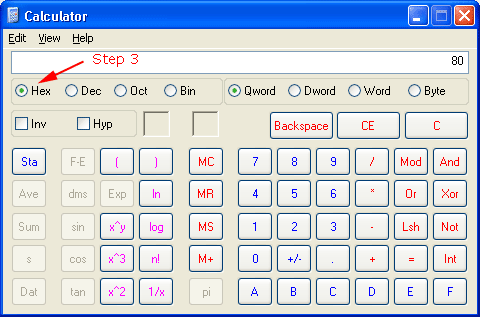If the starting number is in base 2, set the button to bin,
enter the number to convert,
then click to dec, oct, or hex.

If the starting number is in base 16, set the button to hex,
enter the number to convert,
then click to dec, oct, or bin.

If the starting number is in base 8, set the button to oct,
enter the number to convert,
then click to dec, hex, or bin.

Exponents
If you are trying to solve something like 28*28*28=224, and you want to know what 224 is,
Type in 2
then click the x^y button
then type the exponent, 24
then press =, or hit the enter key to calculate